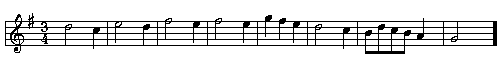The Music of Hailstones Mike Keith Oct 1998

Introduction

The following number "game" is well-known in math circles. Start with any integer greater than 1 and call it s(0). Then generate additional terms of the sequence s(n) by the following rule:

 s(n+1) = s(n)/2 if s(n) is even 3s(n)+1 if s(n) is odd

Variously known as Hailstone numbers (because of their ups and down), the Collatz Problem, or the 3n+1 problem, the central conjecture that every integer eventually reaches the value 1 in its s(n) sequence has never been proved. We now explore the use of the s(n) sequences to produce music.

Music from Hailstone Sequences

An interesting feature, from a musical point of view, is the tension between the two rules for forming the next number in the sequence: the first rule makes the number half as big, but the second rule makes it three times as large. If we look at the sequence produced by some initial n, we see that it tends to go up and down somewhat erratically before (seemingly always) eventually hitting 1. Such a sequence is somewhat is reminiscent of the shape of musical melodies.

Here is an example. The sequence produced by the starting number 30 is

30 15 46 23 70 35 106 53 160 80 40 20 10 5 16 8 4 2 1

To bring this into the range of a musical melody, we pass each number through the following function:

f(n) = [ (10/3) log10(n) ]

where [ ] is the greatest-integer function, yielding the following sequence:

4 3 5 4 6 5 6 5 7 6 5 4 3 2 4 3 2 1 0

These numbers are interpreted as notes in the 7-tone major scale, with 0=root, 1=next note, etc. Note that the fact that the s(n) sequence always ends with 1 means that the last note of a tune produced in this way will always be 0, thus ending on the traditional root of the major scale.

Here are the notes of our tune, written in the key of G and 3/4 time:where the durations of the notes have been chosen by a human (me). If you are equipped to play General MIDI (.mid) files, here is an arrangement of the above tune as a jazz waltz for sax and piano. The first time through the sax player plays the melody exactly as above; the second time there's a bit of improvisation.

Many other interesting tunes can be produced by using this same technique with a different value of s(0).